Next: Integration Up: benches Previous: Non-polynomial systems

• La page de J-P. Merlet
• La page Présentation de COPRIN
• La page "Présentation" de l'INRIA

Subsections

# Optimization

## Branin

Origin: 
Minimize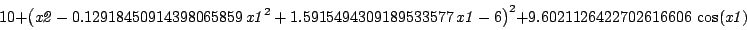forin [-5,10],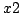in [10,15]

Solutions:: 1 (approximate)
Computation time (September 2006):

 DELL D400 (1.7Ghz) 0.01s

## Goldstein-Price

Origin: 
Let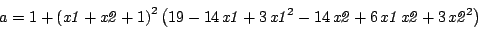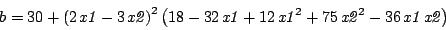and the function to be minimized: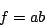where allare in [-2,2]

Solutions:: 1 (approximate)
Computation time (September 2006):

 DELL D400 (1.7Ghz) 0.86s

## Eisenberg

Origin: Maple news group
Maximize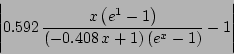for x in the range [0.5,0.8]

Method: Maximize
Solutions:: 1 (approximate)
Computation time (January 2005):

## Klein

Origin:klein@mathematik.uni-kassel.de
Minimize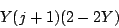subject to the constraint:Range: j=[0,1], Y=[0.01,0.99]

Solutions:: 1 (approximate)
Computation time (April 2003):

## Klein1

Origin:klein@mathematik.uni-kassel.de
Minimizesubject to the constraint: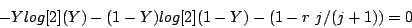Range: j=[0,1], Y=[0.01,0.99], r=[0,1]

Solutions:: 1 (approximate)
Computation time (April 2003):

## MaxCylinder

Origin: COPRIN
Physical meaning: for a robot with 3 degree of freedom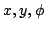a function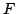defines a singularity variety in which the robot cannot be controlled. We are looking for the maximal disc centered at a given point of coordinates, such that the disc has not intersection with the singularity variety for any orientation in the range [-a,a]. The orientation may thus be written as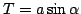and the singularity variety is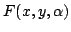, The problem is solved with a Lagrange multiplier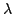by writing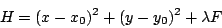The derivatives ofwith respect toare combined to eliminate. The optimality equations constitute then a system of 3 equations in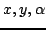. The roots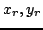of this system are used to calculate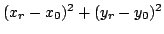and the lowest value of this quantity is the square of the largest singularity-free disc.

The 3 equations to solve are:


-13626.359958*x-17242.663338*y+306.1030*y^2*sin(alpha)^4+1224.4120*y*sin(alpha)^3*x
-327743.551830*sin(alpha)^4-2623771.960340*sin(alpha)^3+561700.662040*sin(alpha)^2
-306.1030*x^2*sin(alpha)^4-1680682.162260*sin(alpha)+182179.510990-1256.4908*x^2*sin(alpha)^3+
1256.4908*y^2*sin(alpha)^3+1256.4908*y*x-48283.435536*x*sin(alpha)^2-16255.087100*y*sin(alpha)^2
+306.1030*x^2-8129.699104*y*sin(alpha)+1224.4120*y*sin(alpha)*x-69790.833024*y*sin(alpha)^3
-306.1030*y^2-1256.4908*sin(alpha)*x^2+129340.554748*sin(alpha)*x+151105.785340*sin(alpha)^3*x+
1256.4908*sin(alpha)*y^2+22752.806830*y*sin(alpha)^4
-1256.4908*y*x*sin(alpha)^4+27004.058342*x*sin(alpha)^4 =0

-4*(x-15)*cos(alpha)*(942.3681*sin(alpha)^2*x*y-19159.084776*x-15238.646187*y+306.1030*y*sin(alpha)^3*x
+40242.36852580*sin(alpha)^3+197386.15916499*sin(alpha)^2-66170.43895050*sin(alpha)+
1857.6978*sin(alpha)^2*x^2+172248.32737589-246.6856*x^2*sin(alpha)^3+381.5598*y^2*sin(alpha)^3+
314.1227*y*x-103723.104768*x*sin(alpha)^2-62039.861505*y*sin(alpha)^2+619.2326*x^2+
24141.717768*y*sin(alpha)-27346.225342*y*sin(alpha)^3+466.1811*y^2-8127.543550*sin(alpha)*x+
8244.982830*sin(alpha)^3*x+1398.5433*sin(alpha)^2*y^2) = 0

-1367.419669*x+6984.263479*y+190.7799*y^2*sin(alpha)^4+628.2454*y*sin(alpha)^3*x+
20121.18426290*sin(alpha)^4+131590.77277666*sin(alpha)^3-66170.43895050*sin(alpha)^2
-123.3428*x^2*sin(alpha)^4+344496.65475178*sin(alpha)+1238.4652*x^2*sin(alpha)^3+
932.3622*y^2*sin(alpha)^3-153.0515*y*x-29221.45558380-8127.543550*x*sin(alpha)^2+
24141.717768*y*sin(alpha)^2+123.3428*x^2-30477.292374*y*sin(alpha)+628.2454*y*sin(alpha)*x
-41359.907670*y*sin(alpha)^3-190.7799*y^2+1238.4652*sin(alpha)*x^2-38318.169552*sin(alpha)*x
-69148.736512*sin(alpha)^3*x+932.3622*sin(alpha)*y^2-13673.112671*y*sin(alpha)^4+
153.0515*y*x*sin(alpha)^4+4122.491415*x*sin(alpha)^4 = 0

the last one being the singularity condition.

Method: GradientSolve+ a specific simplification procedure that allow to avoid determining roots leading to a largest disc than the minimal one already computed
Solutions:: 1
Computation time (August 2004):

 DELL D400 (1.7Ghz) 2s

## O32

Origin: COCONUT
Minimize
37.293239 * x1 + 0.8356891 * x5 * x1 + 5.3578547 * x3^2 - 40792.141

subject to the constraints:
-0.0022053 * x3 * x5 + 0.0056858 * x2 * x5 + 0.0006262 * x1 * x4-6.665593 <= 0
-0.0022053 * x3 * x5-0.0056858 * x2 * x5 - 0.0006262 * x1 * x4 -85.334407 <= 0
0.0071317 * x2 * x5 + 0.0021813 * x3^2 + 0.0029955 * x1 * x2 - 29.48751 <= 0
- 0.0071317 * x2 * x5 - 0.0021813 * x3^2 - 0.0029955 * x1 * x2 + 9.48751 <= 0
0.0047026 *x3 * x5+0.0019085 * x3 * x4 + 0.0012547 * x1 * x3 - 15.699039 <= 0
- 0.0047026 *x3 * x5 - 0.0019085 * x3 * x4 - 0.0012547 * x1 *x3+10.699039 <= 0

Method: Minimize+3B Solutions:: 1
Computation time (August 2004):
 DELL D400 (1.7Ghz) 2.04s

## Rastrigin

Origin: 
Minimize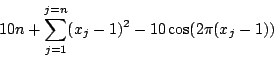forin [-5.12,5.12]

Solutions:: 1 (approximate)
Computation time (September 2006):

 DELL D400 (1.7Ghz) 0.7s (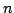=5), 2.07s (=10)

## Rastrigin modified

Origin: 
Minimizeforin [-5.12,5.12]

Solutions:: 1 (approximate)
Computation time (September 2006):

 DELL D400 (1.7Ghz) 0.48s (=5), 2.71s (=10)

## Rosenbrock

Origin: 
Minimizeforin [-5.2,5.2]

Solutions:: 1 (approximate)
Computation time (September 2006):

 DELL D400 (1.7Ghz) 0.85s (=5), 2.22s (=10), 278s (=15)

## Shekel

Origin: 
Letbe:and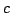:and the function to be minimized: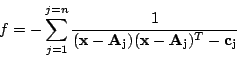whereis therow ofand allare in [0,10]

Solutions:: 1 (approximate)
Computation time (September 2006):

 DELL D400 (1.7Ghz) 0.31s (=5), 1.66s (=7), 2.61s (=10)

## Shubert

Origin: 
Minimizeforin [-10,10],in [-10,10]

Solutions:: 1 (approximate)
Computation time (September 2006):

 DELL D400 (1.7Ghz) 23.3s

## Six hump camel

Origin: 
Minimize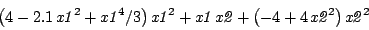forin [-3,3],in [-2,2]

Solutions:: 1 (approximate)
Computation time (September 2006):

 DELL D400 (1.7Ghz) 0.96s

## Tf12

Origin: COCONUT
Minimize
x1+0.5*x2+x3/3.

subject to, for i from 0 to M
-x1-i*h*x2-(i*h)^2*x3+tan(i*h) <= 0

with h=1/M

Method: Minimize+3B Solutions:: 1
Computation time (August 2004):

 DELL D400 (1.7Ghz) 60sNext: Integration Up: benches Previous: Non-polynomial systems
• La page de J-P. Merlet